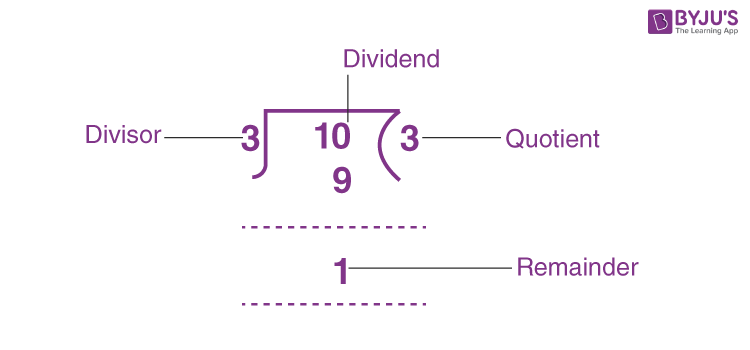# Remainder

Remainder is the value left after the division. If a number (dividend) is not completely divisible by another number (divisor) then we are left with a value once the division is done. This value is called the remainder.

For example, 10 is not exactly divided by 3. Since the closest value we can get is 3 x 3 = 9, which is 1 less than 10.

10 ÷ 3 → 3 R 1, where 3 is the quotient and 1 is the remainder.In arithmetic, division is one of the four major operations performed on integers, real numbers, complex numbers and algebra. The other three arithmetic operations are:

• Subtraction
• Multiplication

## Remainder in Maths

In division, the four main parts are:

• Dividend
• Divisor
• Quotient
• Remainder

A dividend is the number or value that is divided. A divisor is the value that divides the other number. A quotient is the answer we get when one value is divided by another value. The relation between dividend, divisor and quotient is:

Dividend = Divisor x Quotient

Remainder is the number that is left when dividend is not completely divisible by the divisor. Therefore, we can say:

Dividend = Divisor x Quotient + Remainder

Examples are:

• 12 ÷ 5 = 2 Remainder 2 since 5 x 2 = 10 and 10 + 2 = 12
• 33 ÷ 10 = 3 Remainder 3, since 10 x 3 = 30 and 30 + 3 = 33
• 46 ÷ 5 = 9 Remainder 1, since 5 x 9 = 45 and 45 + 1 = 46

## Remainder Formula

As we know:

Dividend = Divisor x Quotient + Remainder

Therefore,

Remainder = Dividend – (Divisor x Quotient)

This is the formula for remainder.

## How to Find Remainder

Finding the remainder is an easy method. We need to just divide the number by another number with its multiples and get the remainder. Let us solve some examples to learn more.

• 43 = 8 x 5 + 3, 3 is the remainder
• 87 = 8 x 10 + 7, 7 is the remainder
• 114 = 7 x 16 + 2, 2 is the remainder

## Frequently Asked Questions on Remainder

### What is remainder in maths?

A remainder is the value that is left after division is completed.

### Give an example of remainder.

When 25 is divided by 4, 1 is the remainder.

### What is the formula for remainder?

The formula to find the remainder is given by:

Dividend/Divisor = Quotient + Remainder/Divisor

### What is the remainder of 17 divided by 5?

17 divided by 5 = 3 R 2

Where 3 is the quotient and 2 is the remainder.

### What is the remainder of 65 divided by 6?

65 ÷ 6 = 10 R 5

Since, 6 x 10 = 60 and adding 5 gives 65